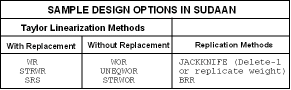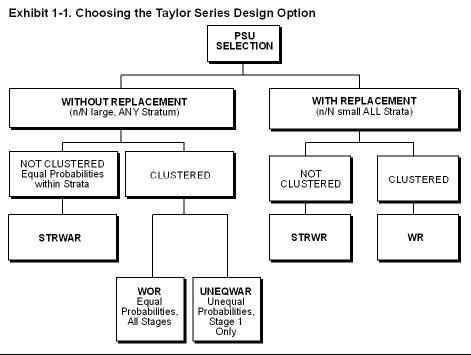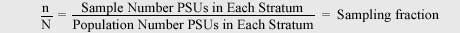## SUDAAN Design Options

Additional information on all design options in SUDAAN can be found in the SUDAAN 11 Language Manual.   A summary of the SUDAAN design options is provided below.

There are eight design options in SUDAAN. Your design choice determines how standard error estimates are obtained from SUDAAN.

In SUDAAN, variance estimation is based on either the Taylor Series Linearization Method (equivalent to GEE in regression procedures) or Replication Methods (Jackknife and BRR). Six of the eight design options supported by SUDAAN compute variance estimates based on Taylor linearization; the other two compute variance estimates based on replication methods. Taylor linearization designs can be further distinguished by whether they assume with-replacement or without-replacement sampling in the first stage of sample selection.

These eight design types allow you to specify a wide variety of sample designs often found in sample surveys and other correlated data situations. Choosing from among these eight design options is the first step in specifying your sample design. The design options available in SUDAAN are the following:Taylor Series Methods

SUDAAN's DESIGN=WR

• Sampling with-replacement at the first stage (or with small sampling fractions--say less than 10%--in every first-stage stratum). The sampling fraction in a first-stage stratum is the number of primary sampling units (PSUs) selected into the sample divided by the population number of PSUs in the stratum.
• Sampling with or without-replacement at subsequent stages.
• Sampling with equal or unequal probabilities of selection at both the first and subsequent stages.

The WR design is the default design in SUDAAN; if you omit the DESIGN= option from your PROC statement, SUDAAN assumes a WR design. For a WR design, SUDAAN estimates the variances using the between-PSU within-stratum variance component. This design is valid when the PSUs are independent (which is implied by with-replacement sampling) and the PSU totals of linearized values can be estimated without bias. In the absence of complete design information, the WR design is often chosen to approximate variances for more complicated designs.

The WR design is used for most nonsurvey applications involving clustered data. It is the most commonly used design option for implementing GEE model-fitting techniques in the regression setting.

SUDAAN's DESIGN=STRWR

• A single-stage design (no clustering).
• Stratified random sampling with-replacement (or small sampling fractions within each stratum).
• Equal or unequal probabilities of selection within each stratum.

SUDAAN's DESIGN=SRS

• A single-stage design (no clustering or stratification).
• Simple random sampling (equal probabilities of selection).
• Small sampling fraction (no finite population corrections needed).

SUDAAN's DESIGN=WOR

• Sampling without-replacement at the first stage (or with large sampling fractions in any first-stage stratum). The sampling fraction in a first-stage stratum is the number of PSUs selected into the sample divided by the population number of PSUs in the stratum.
• Sampling with or without-replacement at subsequent stages. For example, you may want to switch to with-replacement sampling if you have small s ampling fractions within each PSU.
• Sampling with equal probabilities of selection within each stratum and at each stage of without-replacement sampling.
• The WOR design requires knowledge of the population counts in each stratum or PSU at each stage of without-replacement sampling. These population counts are needed because the WOR design computes variances according to a multi-stage formula that computes the finite population correction factors (FPCs) at each stage.

SUDAAN's DESIGN=UNEQWOR

• Sampling without-replacement with unequal probabilities of selection at the first stage.
• Sampling with equal probabilities at subsequent stage(s), with or without-replacement.

SUDAAN's DESIGN=STRWOR

• A single-stage design (no clustering).
• Stratified random sampling without-replacement (or large sampling fractions in at least one stratum).
• Equal probabilities of selection within each stratum.

Choosing the Appropriate Taylor Linearization Method

The matrix below can be used to help to choose from among the six SUDAAN design options using Taylor Linearization.

Without_replacement options (WOR, STRWOR, UNEQWOR) can incorporate with-replacement sampling in some strata, without-replacement sampling in others, or exclusive with-replacement sampling after first stage.

DESIGN=WR is the default variance computation. DESIGN=WR is appropriate for complex sample surveys that are clustered (or multi-stage) and do not require FPCs, and it should be used for GEE applications.Replication Methods

SUDAAN's DESIGN= JACKKNIFE

• Sampling with-replacement at the first stage (or with small sampling fractions--less than 10%--in every first-stage stratum). The sampling fraction in a first_stage stratum is the number of PSUs selected into the sample divided by the population number of PSUs in the stratum.
• Sampling with or without-replacement at subsequent stages.
• Sampling with equal or unequal probabilities of selection at both the first and subsequent stages.

DESIGN=JACKKNIFE is often used in non-survey applications involving clustered data. The most common jackknife method for sample surveys is to delete one PSU (or cluster for the correlated data). The weights for the remaining PSUs in the same stratum are adjusted to account for the deleted PSU. We refer to this as the Delete-1 jackknife method, and it is the default jackknife design in SUDAAN.

If there are many PSUs, it is possible to construct a large number of jackknife replicates in this manner. SUDAAN 8.0 makes this construction possible through the addition of two new sample design statements, JACKWGTS and JACKMULT. We refer to this option as the Replicate Weight jackknife method.

SUDAAN's DESIGN=BRR

• The sample design is specified by the series of replicate weights listed on the REPWGT statement. BRR replicate weights are usually developed under the same assumptions as those listed for WR and jackknife, but special weights may be developed that account for without-replacement sampling. SUDAAN assumes that the replicate weights have already been developed and are available on the input data file.